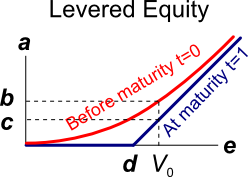# Fight Finance

#### CoursesTagsRandomAllRecentScores

In the Merton model of corporate debt, buying a levered company's debt is equivalent to buying risk free government bonds and:

In the Merton model of corporate debt, buying a levered company's shares is equivalent to:

In the Merton model of corporate debt, buying a levered company's debt is equivalent to buying the company's assets and:

Which of the following is the least useful method or model to calculate the value of a real option in a project?

A risky firm will last for one period only (t=0 to 1), then it will be liquidated. So it's assets will be sold and the debt holders and equity holders will be paid out in that order. The firm has the following quantities:

$V$ = Market value of assets.

$E$ = Market value of (levered) equity.

$D$ = Market value of zero coupon bonds.

$F_1$ = Total face value of zero coupon bonds which is promised to be paid in one year.The levered equity graph above contains bold labels a to e. Which of the following statements about those labels is NOT correct?

A risky firm will last for one period only (t=0 to 1), then it will be liquidated. So it's assets will be sold and the debt holders and equity holders will be paid out in that order. The firm has the following quantities:

$V$ = Market value of assets.

$E$ = Market value of (levered) equity.

$D$ = Market value of zero coupon bonds.

$F_1$ = Total face value of zero coupon bonds which is promised to be paid in one year.The risky corporate debt graph above contains bold labels a to e. Which of the following statements about those labels is NOT correct?

One of the reasons why firms may not begin projects with relatively small positive net present values (NPV's) is because they wish to maximise the value of their:

A moped is a bicycle with pedals and a little motor that can be switched on to assist the rider. Mopeds offer the rider:

You're thinking of starting a new cafe business, but you're not sure if it will be profitable.

You have to decide what type of cups, mugs and glasses you wish to buy. You can pay to have your cafe's name printed on them, or just buy the plain un-marked ones. For marketing reasons it's better to have the cafe name printed. But the plain un-marked cups, mugs and glasses maximise your:

Some financially minded people insist on a prenuptial agreement before committing to marry their partner. This agreement states how the couple's assets should be divided in case they divorce. Prenuptial agreements are designed to give the richer partner more of the couples' assets if they divorce, thus maximising the richer partner's:

An expansion option is best modeled as a or option?

An abandonment option is best modeled as a or option?

A timing option is best modeled as a or option?

According to option theory, it's rational for students to submit their assignments as or as possible?

The cheapest mobile phones available tend to be those that are 'locked' into a cell phone operator's network. Locked phones can not be used with other cell phone operators' networks.

Locked mobile phones are cheaper than unlocked phones because the locked-in network operator helps create a monopoly by:

Your firm's research scientists can begin an exciting new project at a cost of $10m now, after which there’s a: • 70% chance that cash flows will be$1m per year forever, starting in 5 years (t=5). This is the A state of the world.
• 20% chance that cash flows will be $3m per year forever, starting in 5 years (t=5). This is the B state of the world. • 10% chance of a major break through in which case the cash flows will be$20m per year forever starting in 5 years (t=5), or the project can be expanded by investing another $10m (at t=5) which is expected to give cash flows of$60m per year forever, starting at year 9 (t=9). This is the C state of the world.

The firm's cost of capital is 10% pa.

What's the present value (at t=0) of the option to expand in year 5?

A risky firm will last for one period only (t=0 to 1), then it will be liquidated. So it's assets will be sold and the debt holders and equity holders will be paid out in that order. The firm has the following quantities:

$V$ = Market value of assets.

$E$ = Market value of (levered) equity.

$D$ = Market value of zero coupon bonds.

$F_1$ = Total face value of zero coupon bonds which is promised to be paid in one year.

What is the payoff to equity holders at maturity, assuming that they keep their shares until maturity?

A risky firm will last for one period only (t=0 to 1), then it will be liquidated. So it's assets will be sold and the debt holders and equity holders will be paid out in that order. The firm has the following quantities:

$V$ = Market value of assets.

$E$ = Market value of (levered) equity.

$D$ = Market value of zero coupon bonds.

$F_1$ = Total face value of zero coupon bonds which is promised to be paid in one year.

What is the payoff to debt holders at maturity, assuming that they keep their debt until maturity?